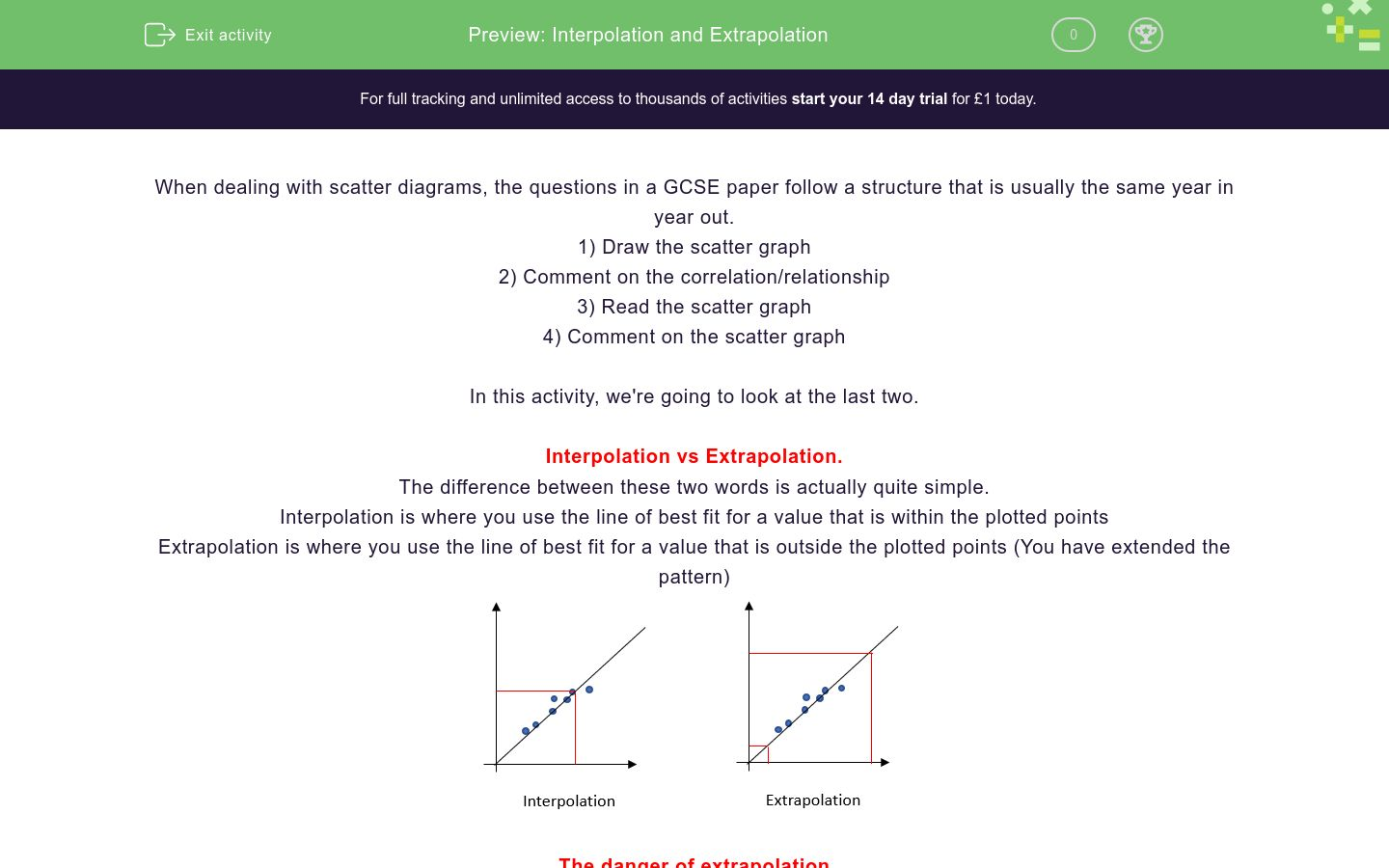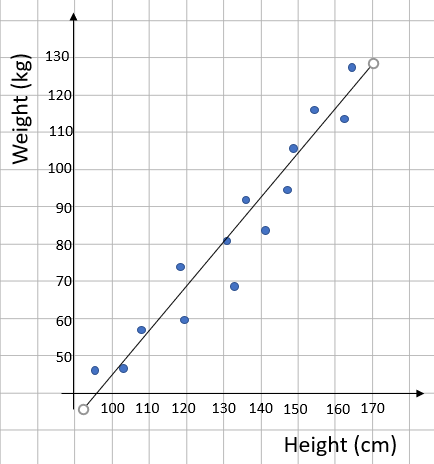# Interpolation and Extrapolation

In this worksheet, students practice interpolation and extrapolation in scatter diagramsKey stage:  KS 4

GCSE Subjects:   Maths

GCSE Boards:   AQA, Eduqas, Pearson Edexcel, OCR

Curriculum topic:   Statistics

Curriculum subtopic:   Statistics, Analysing Data

Difficulty level:### QUESTION 1 of 10

When dealing with scatter diagrams, the questions in a GCSE paper follow a structure that is usually the same year in year out.

1) Draw the scatter graph

2) Comment on the correlation/relationship

4) Comment on the scatter graph

In this activity, we're going to look at the last two.

Interpolation vs Extrapolation.

The difference between these two words is actually quite simple.

Interpolation is where you use the line of best fit for a value that is within the plotted points

Extrapolation is where you use the line of best fit for a value that is outside the plotted points (You have extended the pattern)The danger of extrapolation

When you are drawing a line of best fit, we draw a straight line through the points and use this to read values.

The problem here is we don't actually know what happens outside the points we are given. How do we know that the graph doesn't take a sudden upwards turn? How do we know it doesn't flatten out? or turn downwards?

Because of this, we have to be very wary of using extrapolated values.

How would this be phrased in an exam?

The common way this is phrased would be along the lines of...

'James wants to use this scatter graph to predict the height of a man who weighs 100 kg, explain why this could give a result that isn't accurate.'

The answer to this will always be...

'He is using a value outside of the points that I have plotted'

You don't even have to use the word extrapolated.

Interpolation is...

Extrapolation is...

Is the following prediction Interpolation or Extrapolation?Interpolation

Extrapolation

Is the following prediction Interpolation or Extrapolation?Interpolation

Extrapolation

A scatter graph is drawn to show the relationship between height and weight.Use this scatter graph to predict the weight of a man who weighs 90 kg

(Give your height to the nearest cm)

A scatter graph is drawn to show the relationship between height and weight.Use this scatter graph to predict the weight of a woman who is 1.2 m tall

(Give your weight to the nearest kg)

A scatter graph is drawn to show the value of a car and its age in years.Use this scatter graph to predict the value of a 7 year old car

Give your value to the nearest £100

A scatter graph is drawn to show the value of a car and its age in years.How old is a car that is worth £9000

A scatter graph comparing the average speeds and time taken for 7 journeys is shown.If you want to predict the time taken for a given speed, which speeds could you predict accurately?

10

20

30

40

45

A scatter graph comparing the average speeds and time taken for 7 journeys is shown.If you want to predict the average speed for a given journey time, which times could you not predict accurately?

0.4

0.6

1.1

1.6

2.0

• Question 1

Interpolation is...

EDDIE SAYS
Think of the root of the word Interpolation. Interpolation = Inside
• Question 2

Extrapolation is...

EDDIE SAYS
Think of the root of the word Extrapolation Extrapolation = Extend You must extend the line of best fit, you are outside the plotted points.
• Question 3

Is the following prediction Interpolation or Extrapolation?Interpolation
EDDIE SAYS
The prediction is inside the plotted points. This means it is ...
• Question 4

Is the following prediction Interpolation or Extrapolation?Extrapolation
EDDIE SAYS
The prediction is outside the plotted points. This means it is ...
• Question 5

A scatter graph is drawn to show the relationship between height and weight.Use this scatter graph to predict the weight of a man who weighs 90 kg

(Give your height to the nearest cm)

145
146
147
148
149
EDDIE SAYS
This point is well inside the points so we can do this. If we draw a line from 90 on the weight to the line of best fit and go down to the height axis, we hit at around 147 cm Don't forget that as you are reading from a graph, you will get a margin of error. In this case, you can have an answer between 145 and 149
• Question 6

A scatter graph is drawn to show the relationship between height and weight.Use this scatter graph to predict the weight of a woman who is 1.2 m tall

(Give your weight to the nearest kg)

67
68
69
EDDIE SAYS
This point is well inside the points so we can do this. If we draw a line from 120 on the height to the line of best fit and go across to the weight axis, we hit at around 68 kg Don't forget that as you are reading from a graph, you will get a margin of error. In this case, you can have an answer between 67 and 69
• Question 7

A scatter graph is drawn to show the value of a car and its age in years.Use this scatter graph to predict the value of a 7 year old car

Give your value to the nearest £100

2800
2900
3000
3100
3200
EDDIE SAYS
This point is well inside the points so we can do this. If we draw a line from 7 on the age to the line of best fit and go across to the value axis, we hit at around 3 (this represents £3000) Don't forget that as you are reading from a graph, you will get a margin of error. In this case, you can have an answer between 2800 and 2900
• Question 8

A scatter graph is drawn to show the value of a car and its age in years.How old is a car that is worth £9000

4
EDDIE SAYS
This point is well inside the points so we can do this. If we draw a line from 9 (which represents £9000) on the value to the line of best fit and go down to the value axis, we hit at exactly 4 years Because we hit the value 4 exactly, you don't get a margin of error for this one.
• Question 9

A scatter graph comparing the average speeds and time taken for 7 journeys is shown.If you want to predict the time taken for a given speed, which speeds could you predict accurately?

30
40
EDDIE SAYS
The smallest speed we have plotted it 25 mph and the largest is 42 mph. Any values within these two are interpolated values as they are within the limits of the pattern,
• Question 10

A scatter graph comparing the average speeds and time taken for 7 journeys is shown.If you want to predict the average speed for a given journey time, which times could you not predict accurately?

0.4
1.6
2.0
EDDIE SAYS
The smallest time we have plotted is 0.5 hours and the largest is 1.5 hours Any values outside of these two are extrapolated values as they are outside the limits of the pattern,
---- OR ----

Sign up for a £1 trial so you can track and measure your child's progress on this activity.

### What is EdPlace?

We're your National Curriculum aligned online education content provider helping each child succeed in English, maths and science from year 1 to GCSE. With an EdPlace account you’ll be able to track and measure progress, helping each child achieve their best. We build confidence and attainment by personalising each child’s learning at a level that suits them.

Get started